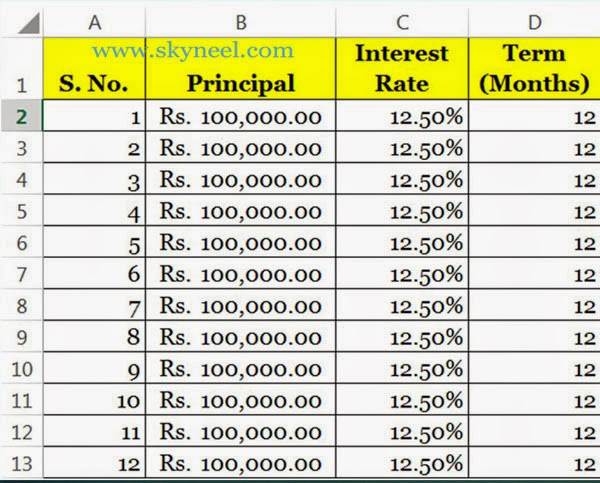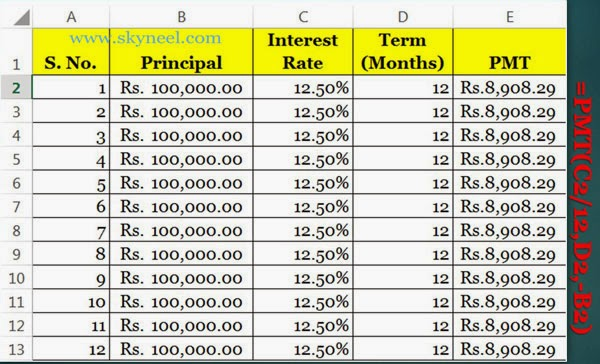# How to use PMT Function in Excel

calculate payments for a loan or the future value of an investment by Excel PMI (Payment Monthly Installment) function by PMT Function in Excel.

If anybody want to take loan from any govt. authorized bank or any private bank he must need to know what is the current annual interest rate is charged by the bank against the loan for a specific period and specific loan amount. PMT function is used to returns the monthly installment amount against the payment of a loan which is based on the specific periodic, and a fixed interest rate.

It is very important to know how you can easily calculate monthly installment amount against to pay any loan with interest amount in MS Excel? Answer of this solution is you can use PMT() which is the most popular financial function of Microsoft Excel. PMT Stands for Payment Monthly Installment. We already discuss about calculate the future value of funds in MS Excel with Future Value (FV) function in previous post.

### Syntax of PMT Function:

pmt(rate,nper,pv,[fv],[type])
rate: Annual Interest Rate
nper: Number of Period
pv: Present/Principal Value
fv: Future Value (It will be optional). If fv is not given then it is expected to be zero, that means the future value of a loan is 0.
type: (It will be optional) If you feed 0, it means end of the
period and if you feed 1, it means beginning of the period. If you ignore this
part then it is assumed to be 0.

For Example:

If you want to take loan Rs. 1,00,000/- for the duration of 12 months @ 12.50% annual interest rate then how could you calculate the installment amount of each month including interest amount in the principal or loan amount.

### Steps how to calculate PMT Function in MS Excel

Step 1: Prepare the following sheet in MS Excel.Step 2: Type the following formula in Cell E2. You have to divide annual interest rate by 12 to calculate monthly interest rate. =PMT(C2/12,D2,-B2). When you press enter key after typing the formula, excel displays Rs. 8,908.29/- installment amount of first month.

Step 3: Now copy this formula in range E3:E13. When you calculate total of the range E2:E13 you will get following result Rs. 1,06,899.44.I hope you like this post and also sort out your problem regarding to PMT Function in Excel. Share this post in your friends and improve your knowledge. Give your important suggestions in the comment box. Thanks.